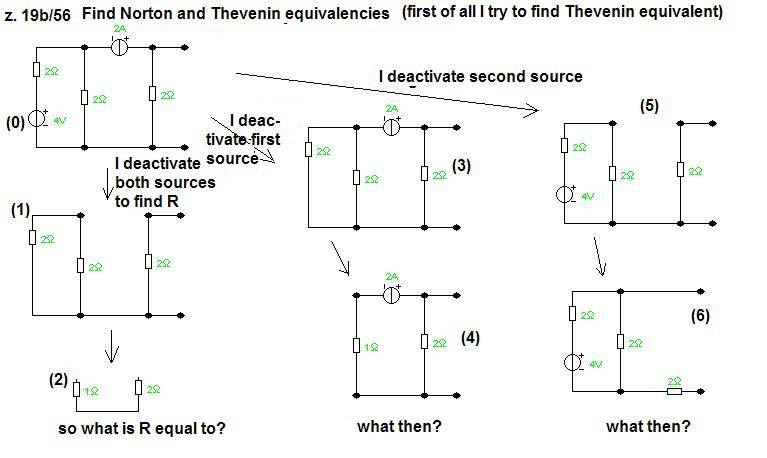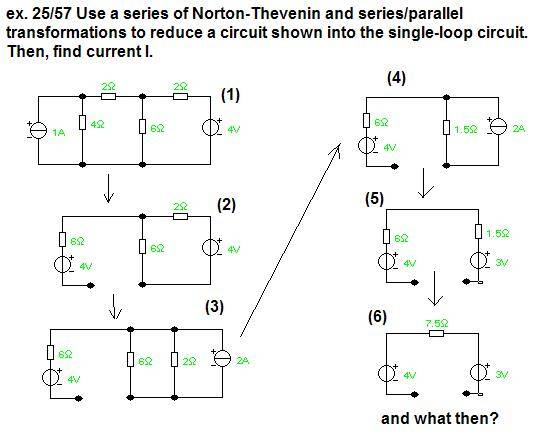# Norton/Thevenin equivalents

## Homework Statement

1) Find Norton and Thevenin equivalents.
2) Use a series of Norton-Thevenin and series/parallel transformations to reduce a circuit shown into the single-loop circuit. Then, find current I. (Current I is between two nodes at the botom of (1) circuit!)

no equations

## The Attempt at a Solution

My way of solution is presented on these pictures.

I have got two exercises which I try to solve.cepheid
Staff Emeritus
Gold Member
For the first exercise, steps 1 and 2 look alright. The equivalent resistance is the equivalent resistance between the terminals (the resistance a load circuit would be exposed to if it were connected between the terminals). As a result, since out of the two resistors you have left, only the 2 Ω resistor is actually between the terminals (the 1 Ω is just dangling), the equivalent resistance is just 2 Ω.

So, the method of finding Rthev was correct. However, I am not sure what method you were using in steps 3, 4, 5, and 6. As far as I know, the Thévénin voltage is just the open circuit voltage, which you can calculate directly in this example. It's just the 2 A of current from the current source going through the rightmost resistor, so that 2 A * 2 Ω = 4 V.

Once you have the Thévénin equivalent, you can get the Norton. It's just Ithev = Vthev / Rthev = 4 V / 2 Ω = 2 A.

This is also obvious because the short circuit current (the current you'd measure across the leads if you shorted them) is 2 A (from the current source)

Last edited:
I thought that Thevenin equivalence can be obtained if I at first deactivate ideal voltage source (3), simplify new circuit and calculate voltage, at second deactivate ideal current source (5), simplify new circuit and calculate voltage. Then add these two voltages and it will be Thevenin voltage. (Analogously I can calculate two currents and add them). But I do not know how to simplify (6) and (7).
What about the second example (Current I is between two nodes at the botom of (1) circuit!)?